Synergetics. Etudes 70.

Is Dedicated to Centennial of Burthday of Outstanding Scientist, Professor

Basin Abram M.

Basina G. I., Basin M. A.

SIC ”Synergetics” of Saint-Petersburg Association of Scientist and Scholars.

Etude 1.

Bifurcations of Exponent of Circumference. Numbers of Basin.

21 October 2009

Phase trajectories of linear dynamically systems are often represent closed cycles, near to the circumferences. In complex field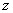circumference with radius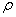may be described with formula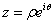, where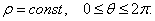Most typical nonlinear problem, yielding to analytically solving, is the problem of determining of dynamics of system, logarithms of which dynamic parameters are subordinated to lineal regularities. Shall we call as exponent of circumference the closed curve in complex plane, which ensues as reflection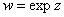. Shall we divide in the last formula the real part from imaginary part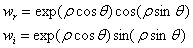.

Shall we find the points of crossing of exponents of circumference with real axis. Condition of there finding may be written in the form: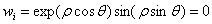. From here follows, that at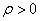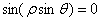. Last equation has counting multitude of decisions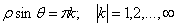. From here we receive: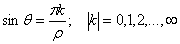. Decisions of last equation subordinate to the restriction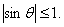or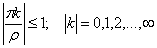.

If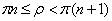, then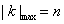. Real coordinate of exponent of circumference at the points of crossing with real axis determines for the next formulas:

Если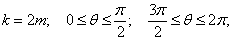то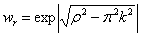.

If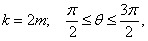then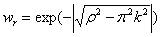.

If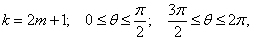then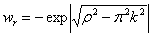.

If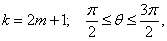then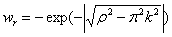.

If we shall consider parametric family of exponents of circumference, depending from parameter, then meanings of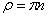are bifurcation meanings. If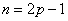, then at the achieving ofappear “from nothing” two negative meanings of points of crossing of exponent of circumference with abscissa (radicals) in the area of spiral numbers, which may be identify in the area of algebraic complex numbers with -1. Later at the increasing ofthese radicals split and go away from -1, one to the right, another –to the left. So, appear and then slide four (two double) radicals. This process continues till thenachieves the value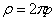. Then appears new bifurcation: at the area of spiral complex numbers appear two new radicals, having at the area of algebraic complex numbers the same meaning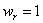. Then occurs splitting of each of these radicals on two, which go away from 1 at different sides. Explanations of these “tricks” may be found, if we shall deduce the character of changing of exponent of circumstance depending from parameterin the complex plane. Shall we consider the dynamics of the point, corresponding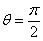. We have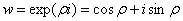. That means that this point moves with the changing ofon the circumference with the  radius equal to 1, rotating then more quickly as more is the value of. This circumference crosses abscissa at the points  -1 and +1. That why new radicals form namely in these points. So the meanings of abscissas of exponents of circumference at the points of crossing with real axis determine for the next formulas:

Ifthen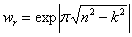.

Ifthen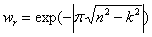.

Ifthen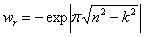.

Ifthen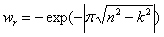.

The numbers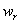, which are the combinations of transcendent and algebraic irrational numbers, are the universal no dimensionally numbers, which characterize bifurcation dynamics of system of exponents of circumference.

On the honor of Centennial of Birthday of outstanding scientist, professor Abram M. Basin we called them Numbers of Basin.

As the universality of fulfilled analysis, we consider, that these numbers in one or another form must be found at the investigation of nonlinear dynamically systems.